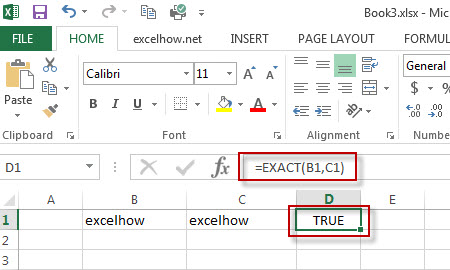# Excel Exact Function

This post will guide you how to use Excel EXACT function with syntax and examples in Microsoft excel.

### Description

The Excel EXACT function compares if two text strings are the same and returns TRUE if they are the same, Or, it will return FALSE.

The EXACT function is a build-in function in Microsoft Excel and it is categorized as a Text Function.

Note: The EXACT function is case-sensitive.

The EXACT function is available in Excel 2016, Excel 2013, Excel 2010, Excel 2007, Excel 2003, Excel XP, Excel 2000, Excel 2011 for Mac.

### Syntax

The syntax of the EXACT function is as below:

`= EXACT (text1,text2)`

Where the EXACT function arguments are:

• Text1 and Text2 -This is a required argument.  Two text strings that you want to compare

### Example

The below example will show you how to use Excel EXACT Text function to check whether the two text string values are identical.

#1 To compare if the text strings in both B1 and C1 cells are the same, just using formula: =EXACT(B1,C1).### More Excel EXACT Function Examples

• Highlight Cells That End with Specfic Characters
If you want to highlight cells that end with a specific text string, you can create a simple formula based on the COUNTIF function to apply conditional formatting. ……
• Sum Cell Value with Case Sensitive
Assuming that you have a list of data in the range A1:B5, and you want to sum cell values based on “excel” product only, and ignore “Excel”. So you can create a new formula based on the SUMPRODUCT function and the EXACT function.…
• Check If Multiple Cells are Equal In Excel
Assuming that you have a list of data in range A1:C1, and you want to compare if these cells are equal, if so, then return True, otherwise, return False. You need to create an Excel array formula based on the AND function and the EXACT function…